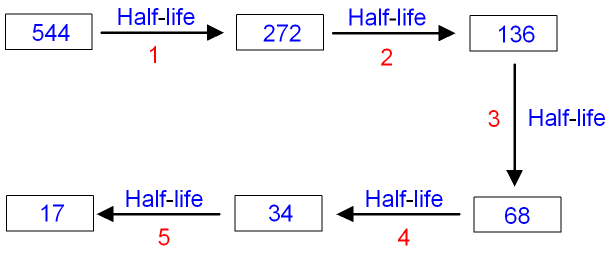gcsescience.com                                       18                                       gcsescience.com

Radioactivity

Using Half-life to Calculate the Count Rate.

Calculations using Half-life.

There are two types of calculation using half-life.

1. If you are given two count rates and you know
how long it takes to get from one to the other,
then you can calculate the half-life of the material.
The method for this is shown on the next page.

2.  If you know the half-life of a material, you can
calculate what the count rate will be at some time in the future.
The method for this is shown below.

How Half-life can be used to Calculate the Count Rate.

Question.
The half-life of a material is 3 hours.
If the initial count rate is 544 Bq,
what will the count rate be after 15 hours?

Answer.
Put the count rate in boxes and use arrows to represent the half-life.

We are told that the half-life of the material is 3 hours.
In 15 hours there are 15 ÷ 3 half-lives
= 5 half-lives.
After each half-life the count rate is halved.After five half-lives the count rate is 17 Bq.

gcsescience.com     Physics Quiz     Index     Radioactivity Quiz     gcsescience.com

Copyright © 2015 gcsescience.com. All Rights Reserved.